## Irregular, but not randomIn a previous post I showed some examples of irregular polyhedra that I’ve been making out of paper. These polyhedra were all based on points distributed quasi-randomly over a sphere. At each point, my program placed a plane tangent to the sphere. The result of the intersection of all of those planes was an irregular polyhedron.

The 47-hedron in the picture above was made in a rather different way. It’s certainly irregular, but the process that created it was not at all random. As in the previous polyhedra, the starting point was a sphere with a number of points distributed over it. But this time, the points were placed according to a simple and perfectly regular rule.

Imagine you you draw a line from one pole of a sphere to the opposite pole, winding sort-of-helically around the sphere. Then place a number of points at exactly equal spacings along this line. In the examples below, there are 43 points.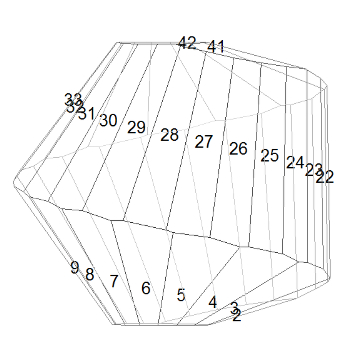If your quasi-helix had 2.5 turns, the result would look like the diagram on the right. The numbers are where the points are placed on the imaginary sphere; the resulting polyhedron is also drawn. The helical structure is very clear, but notice the irregularity of the faces: for example, the lower edges of faces 26, 27, and 28 are all different. This is because the radius of the quasi-helix is not constant, so turns of the helix near the poles will have fewer faces on them than turns near the equator, which means that the way the numbers on one turn line up with the numbers on the previous and subsequent turns keeps changing.

If we increase the number of turns in the quasi-helix to 5, the helical structure is still clear: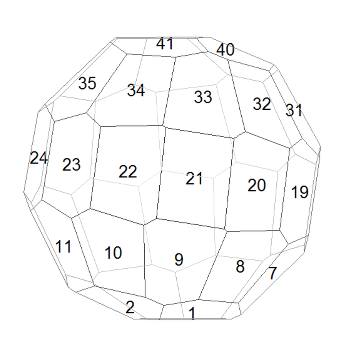With 7.5 turns in the quasi-helix (below), adjacent-numbered faces are now so far apart that the faces on the turns above and below them are starting to intrude into the spaces between them. See, for example, how faces 27 and 28 are almost pushed apart by faces 19 and 35.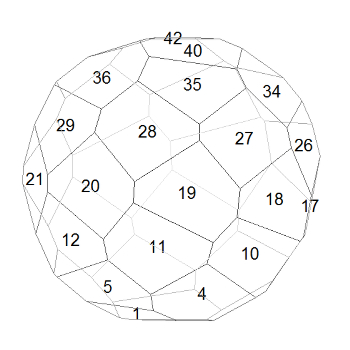By the time we have 10 turns (below), consecutively-numbered faces are so far apart that faces from the turns above and below often meet between them, making the helical structure hard to discern (see, for example, how faces 34 and 22 squeeze in between faces 28 and 29). The polyhedron is beginning to look quite irregular, but note that consecutively-numbered faces are still more similar to each other than they are to the other faces.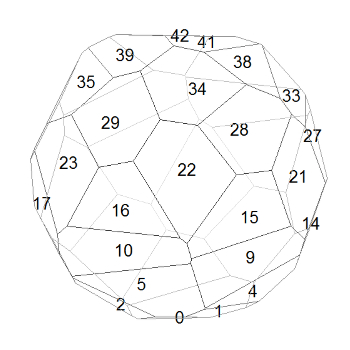The 47-hedron on the right (same as at the top of the post) was based on a quasi-helix with about 12.5 turns. At first sight, it looks quite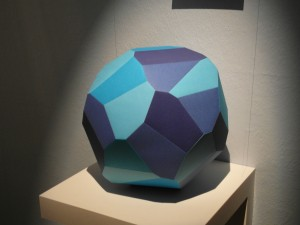random, and it’s certainly irregular, but there are still visible similarities between faces if you look hard enough. Compare, for example, the mid-blue and light-blue faces at roughly 10 o’clock and 2 o’clock respectively. And what about the dark-blue and very dark-blue faces just below the centre? One looks suspiciously like a rotated version of the other – and it is! In fact the whole polyhedron has an axis of 180° rotational symmetry. This suprised me when I spotted it, but it shouldn’t have. A helix has 180° rotational symmetry (turn a corkscrew upside down and it looks the same) so it’s inevitable that shapes based on a helix will have that symmetry also.